Visitors Online: 77 | Tuesday 16th July 2019
 CBSE Guess > Papers > Question Papers > Class XII > 2005 > Maths > Compartment Outside Delhi Set-II MATHS 2005 (Set II—Compartment Outside Delhi)
Expect for the following questins, all the remaining question have been asked in Set I. SECTION - A Q. 1. Using properties of determinants, show thatQ. 5. Evaluate:Q. 10. If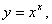show that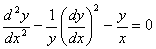Q. 13. Using differentials, find the approximate value of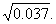Q. 18. Using integration, find the region in the first quadrant enclosed by the x-axis, the line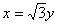and the circle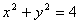. EXERCISE B Q. 19. If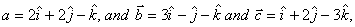then verify that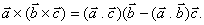Q. 22. A particle just clears wall of height 'b' at a distance 'a' and strikes the ground at a distance 'c' from the point of projection. Prove that the angle of projection is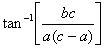and the velocity of projection u is given by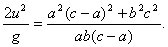Q. 24. Two forces of magnitude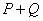and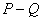make an angle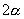with one another, and their resultant makes an anglewith the bisector of the angle between them. Show that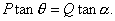SECTION - C Q. 21. Two cards are drawn successively with replacement from a well- shuffled pack of 52 cards. Find the mean and variance for the number of aces. Or There are 5% defective bulbs of bulbs. What is the probability that a sample of 10 bulbs. Will includes not more than 1 defective bulb? (Use e -0.5 = 0.6065) Q. 22. Two bags A and B contain 2 white, 4 red; and 3 white, 3 red balls respectively. One of the bags is selected at random and a ball is drawn from it. If the selected ball is of white colour, find the probability that it is drawn from bag A. Q. 25. A and B are partners sharing profit and losses in the ratio 1 : 2 respectively. They admit C as a new partner, the new profit sharing ratio being 1 : 2 : 2 between A, B and C, respectively. C pays Rs.12,000 as premium for goodwill. How will the premium be shared between A and B? Maths 2005 Question Papers Class XII Delhi Outside Delhi Compartment Delhi Compartment Outside Delhi Set I Set I Set I Set I Set II Set II Set II Set II Set III Set III CBSE 2005 Question Papers Class XII